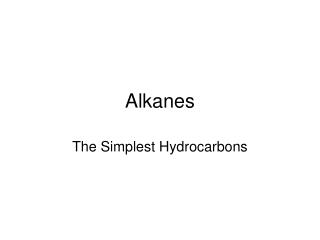DownloadDownload PresentationAlkanes

# Alkanes

Download Presentation## Alkanes

- - - - - - - - - - - - - - - - - - - - - - - - - - - E N D - - - - - - - - - - - - - - - - - - - - - - - - - - -
##### Presentation Transcript

1. Alkanes The Simplest Hydrocarbons

2. Learning outcomes Define and recognise simple alkanes. Write the correct chemical formula of an alkane represented by a structural formula. Given named examples, draw diagrams and write the formulae of simple alkanes. Recall and use the formula of an alkane, when n is given. Keywords Hydrocarbon, alkane, saturated, formula.

3. What is the simplest compound that can be made using one carbon atom and some hydrogen atoms?

4. METHANE The methane molecule is saturated What would the formula for methane be?

5. ETHANE Formula?

6. PROPANE BUTANE Formula? Ball and stick diagram?

7. Can you write a general formula for alkanes? If number of carbon atoms is n then formula = ?

8. Extra info. Double (and triple) bonds are possible. More H atoms could be added so this molecule is NOT saturated This hydrocarbon contains an oxygen atom also It is one of my favourites The alkanes we have looked at are linear. There are also branched alkanes and other possibilities.

9. What is a hydrocarbon? • What is an alkane? • Pentane is an alkane with 5 carbon atoms. Draw a ball and stick model to show its structure and write its formula. • What does a saturated molecule not contain? • What is an important use for alkanes? • 6. Here is an equation for the combustion of methane • CH4 + 2O2 CO2 + 2H2O • What are the reactants? • What are the products? • Is this equation balanced? Explain.